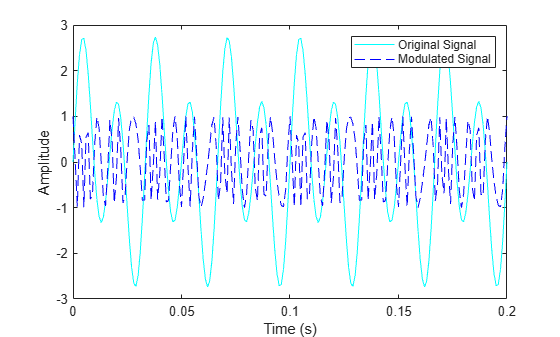Documentation

This is machine translation

Mouseover text to see original. Click the button below to return to the English version of the page.

fmdemod

Frequency demodulation

Syntax

```z = fmdemod(y,Fc,Fs,freqdev) z = fmdemod(y,Fc,Fs,freqdev,ini_phase) ```

Description

`z = fmdemod(y,Fc,Fs,freqdev)` demodulates the modulating signal `z` from the carrier signal using frequency demodulation. The carrier signal has frequency `Fc` (Hz) and sampling rate `Fs` (Hz), where `Fs` must be at least `2*Fc`. The `freqdev` argument is the frequency deviation (Hz) of the modulated signal `y`.

`z = fmdemod(y,Fc,Fs,freqdev,ini_phase)` specifies the initial phase of the modulated signal, in radians.

Examples

collapse all

Set the sample rate and carrier frequency. Generate a time vector having a duration of 0.2 s.

```fs = 1000; fc = 200; t = (0:1/fs:0.2)';```

Create a sinusoidal signal.

`x = sin(2*pi*30*t)+2*sin(2*pi*60*t);`

Set the frequency deviation to 50 Hz.

`fDev = 50;`

Frequency modulate `x`.

`y = fmmod(x,fc,fs,fDev);`

Demodulate `z`.

`z = fmdemod(y,fc,fs,fDev); % Demodulate both channels.`

Plot the original and demodulated signals.

```plot(t,x,'c',t,z,'b--'); xlabel('Time (s)') ylabel('Amplitude') legend('Original Signal','Demodulated Signal')```The demodulated signal is well aligned with the original.